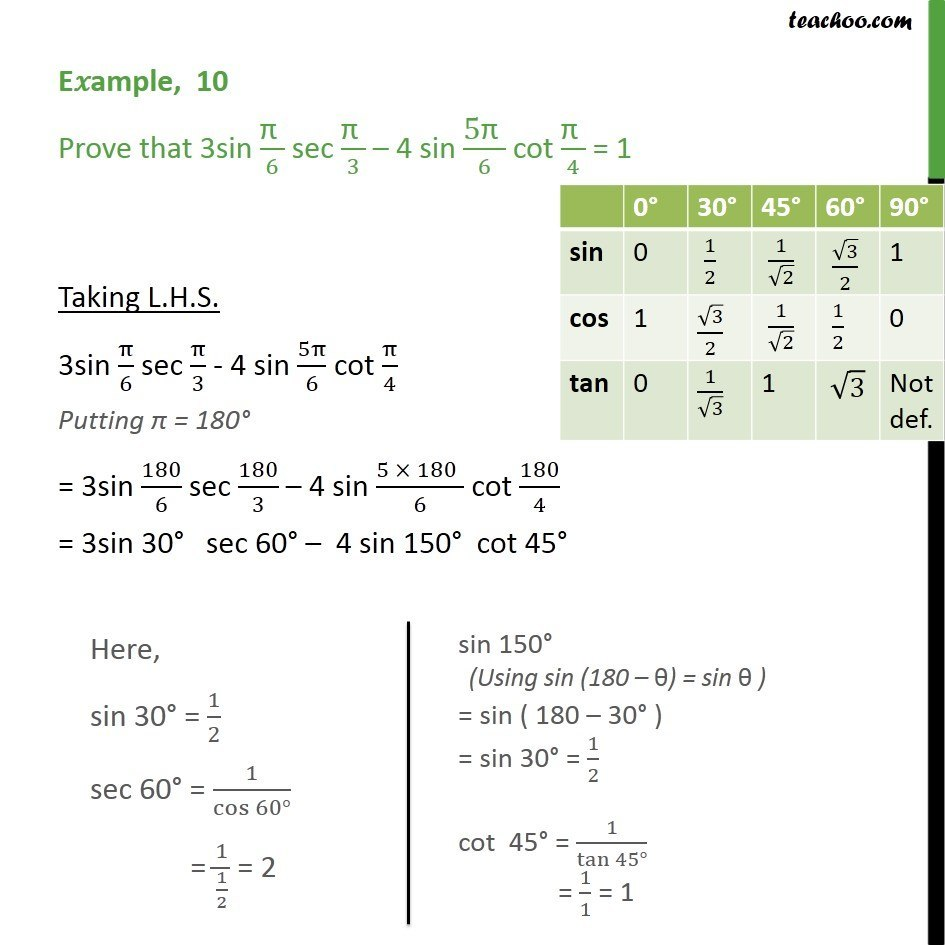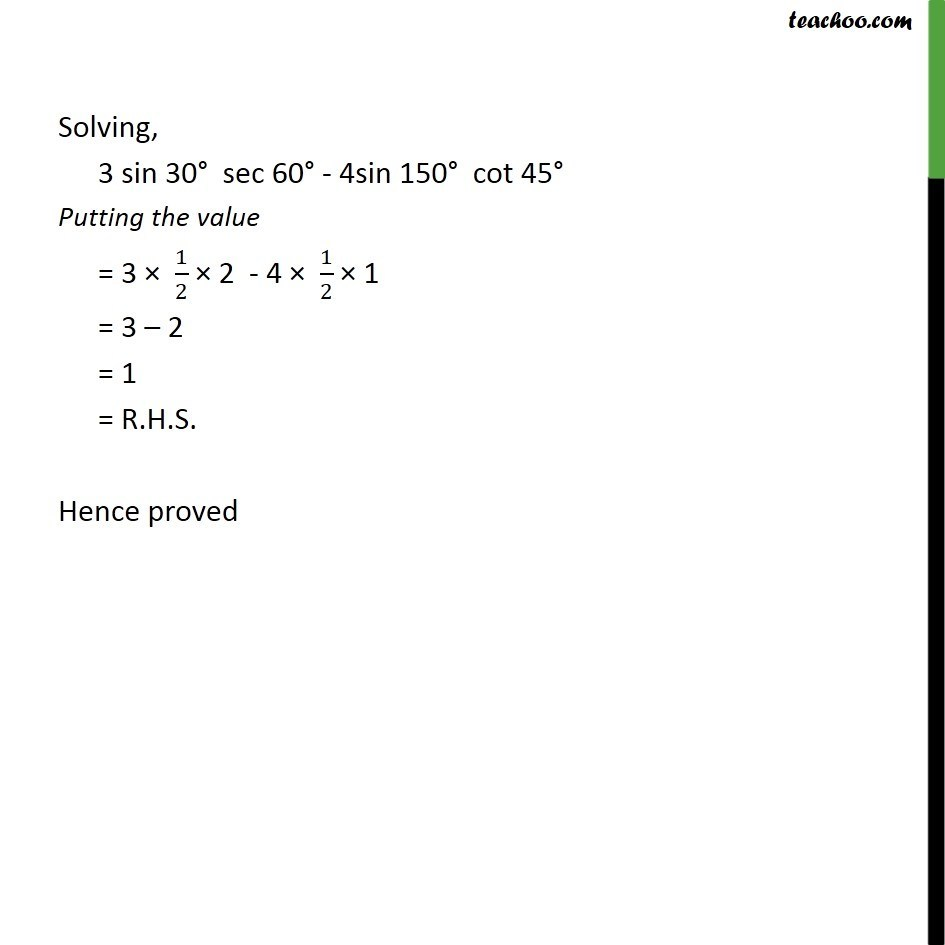Subscribe to our Youtube Channel - https://you.tube/teachoo

1. Chapter 3 Class 11 Trigonometric Functions
2. Concept wise
3. Finding Value of trignometric functions, given angle

Transcript

E ample, 10 Prove that 3sin /6 sec /3 4 sin 5 /6 cot /4 = 1 Taking L.H.S. 3sin /6 sec /3 - 4 sin 5 /6 cot /4 Putting = 180 = 3sin 180/6 sec 180/3 4 sin (5 180" " )/6 cot 180/4 = 3sin 30 sec 60 4 sin 150 cot 45 Solving, 3 sin 30 sec 60 - 4sin 150 cot 45 Putting the value = 3 1/2 2 - 4 1/2 1 = 3 2 = 1 = R.H.S. Hence proved

Finding Value of trignometric functions, given angle